# Chapter 4 - Functions, calling library functions, reading input, string concatenation¶

## The idea of a function¶

You have probably seen the idea of a “function” in math classes. A function is just a rule for computing something. Your pocket calculator has many functions built in for computing powers and square roots and so on. You enter an argument, say 25, and press the button for the square root function, and the result appears on the display. You can use the square root function without really knowing how the result is calculated. We sometimes think of a function as a “black box” – you put a value in, some magic happens, and a result comes out.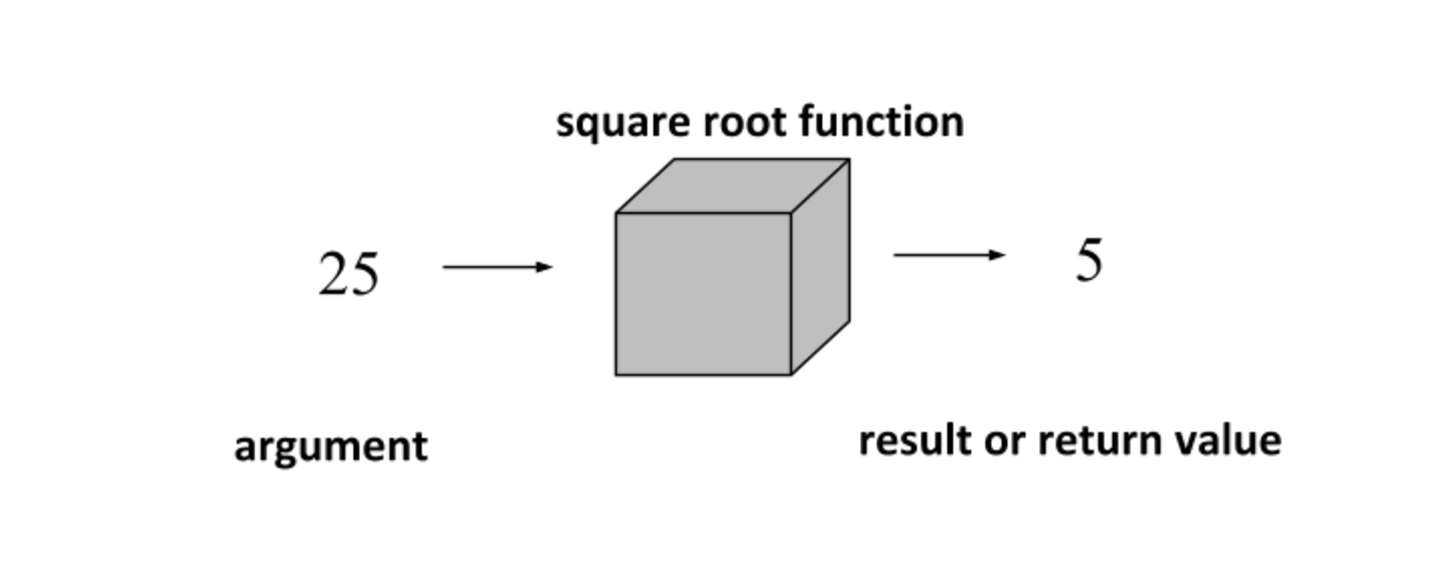The value that you provide as an “input” to the function is called the argument. The result that you get as “output” will be called the return value.

Some functions need more than one argument. For example, there is a simple function for the the area of a rectangle. In order to get the area, you have to provide the rectangle’s length and width.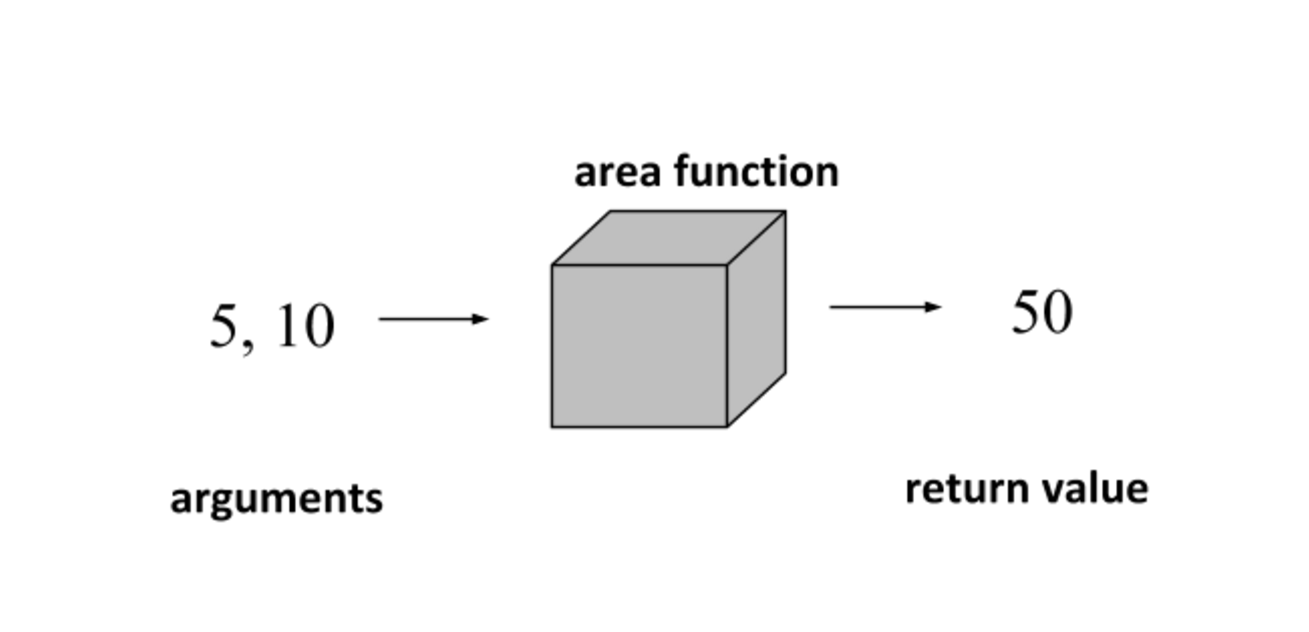We know that we can compute the area of a rectangle with the simple formula length times width, but a function doesn’t have to come from a simple formula, and it doesn’t even have to be mathematical. For example, at the end of the course, your instructor will take your average score and determine a letter grade, a function that probably seems truly mysterious.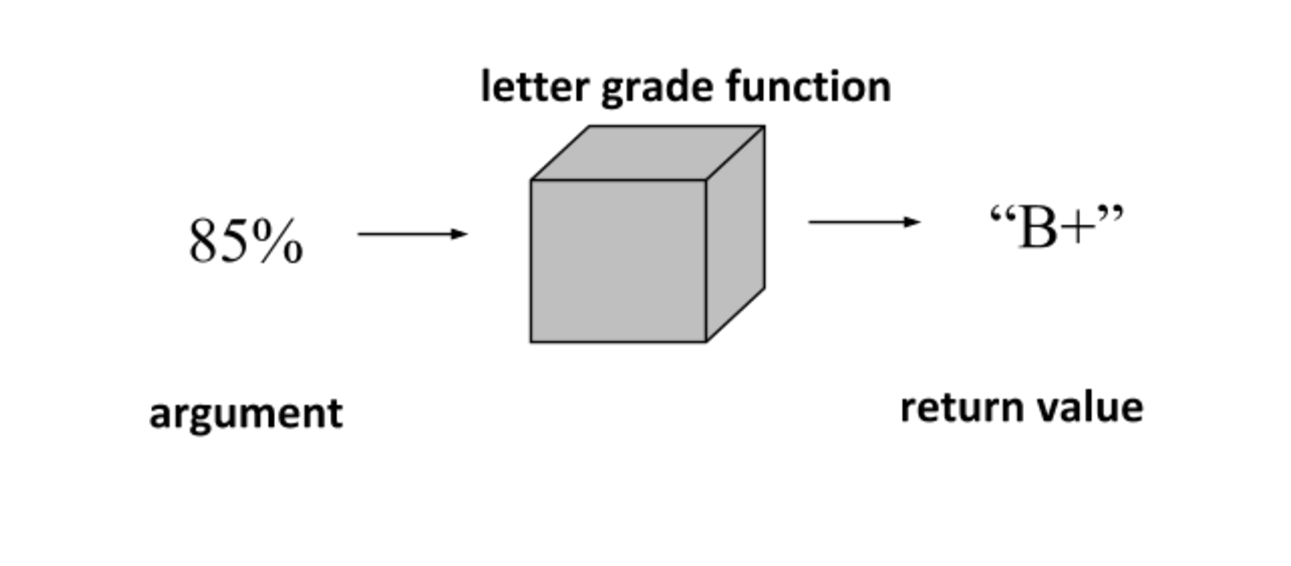## Functions in Python¶

Like a calculator, Python has some built-in functions. Let’s look at a few of them. One example is the string length function, which is called len.

The len function requires one argument, which is a string of text. The return value is the string’s length, that is, the number of characters in the string.

Another example is the built-in function for calculating powers such as “4 to the power 3,” or 4 * 4 * 4 (which is 64) or “2 to the power 5,” which is 32. Computing a power requires two arguments, a base and an exponent. In Python this function is called pow:

So one thing you might notice is that in a math book, a function can have special notation, like the special “square root” symbol √ . But in Python, every function has to have a name which is a valid Python identifier, like the name of a variable.

When we write something like

pow(2, 5)

this expression is known as a function call or function invocation. You write the function’s name, followed by the arguments in parentheses. If there is more than one argument, they are separated by a comma.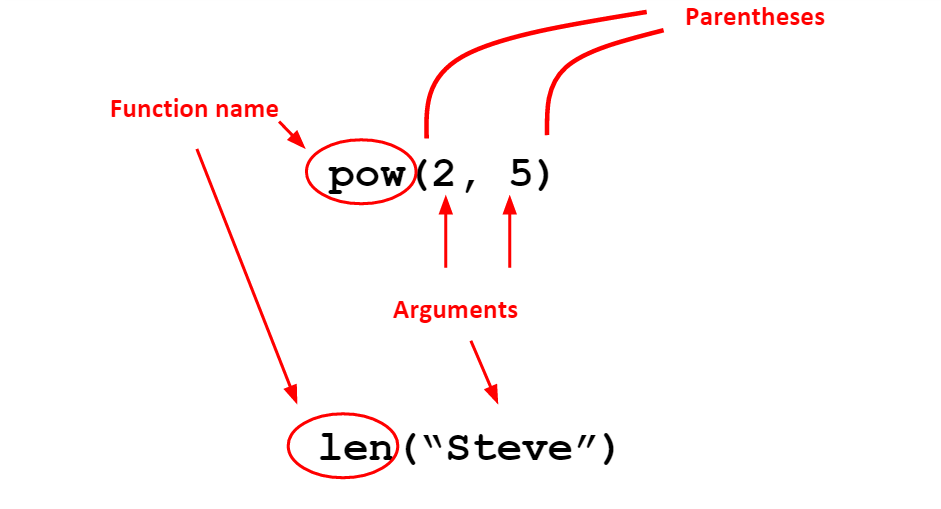The arguments can be any valid expressions of the right type, for example, we could write

pow(1 + 1, 3 + 2)

and the value is still 32. Does the order of the arguments matter? Let’s try this:

which gives us 5 to the power 2, not 2 to the power 5. So the order of the arguments is important. What happens if we don’t provide enough arguments? Try this:

The interpreter is telling us that the pow function expects “at least 2” arguments.

For a function like pow, the function call is an expression with a value that you can use. For example, you could write

That is, the function call pow(2, 5) is really just an expression with the value 32. Function calls can be composed just like other kinds of expressions, for example, len(“Steve”) is 5, so we can compute “2 to the power len("Steve")”:

It is worth noticing that we can call a function without knowing how it works. That’s why the “black box” analogy makes sense. Later we will start writing our own functions and we’ll learn in detail how they work. For now, a perfectly good explanation is that the results are calculated by gnomes.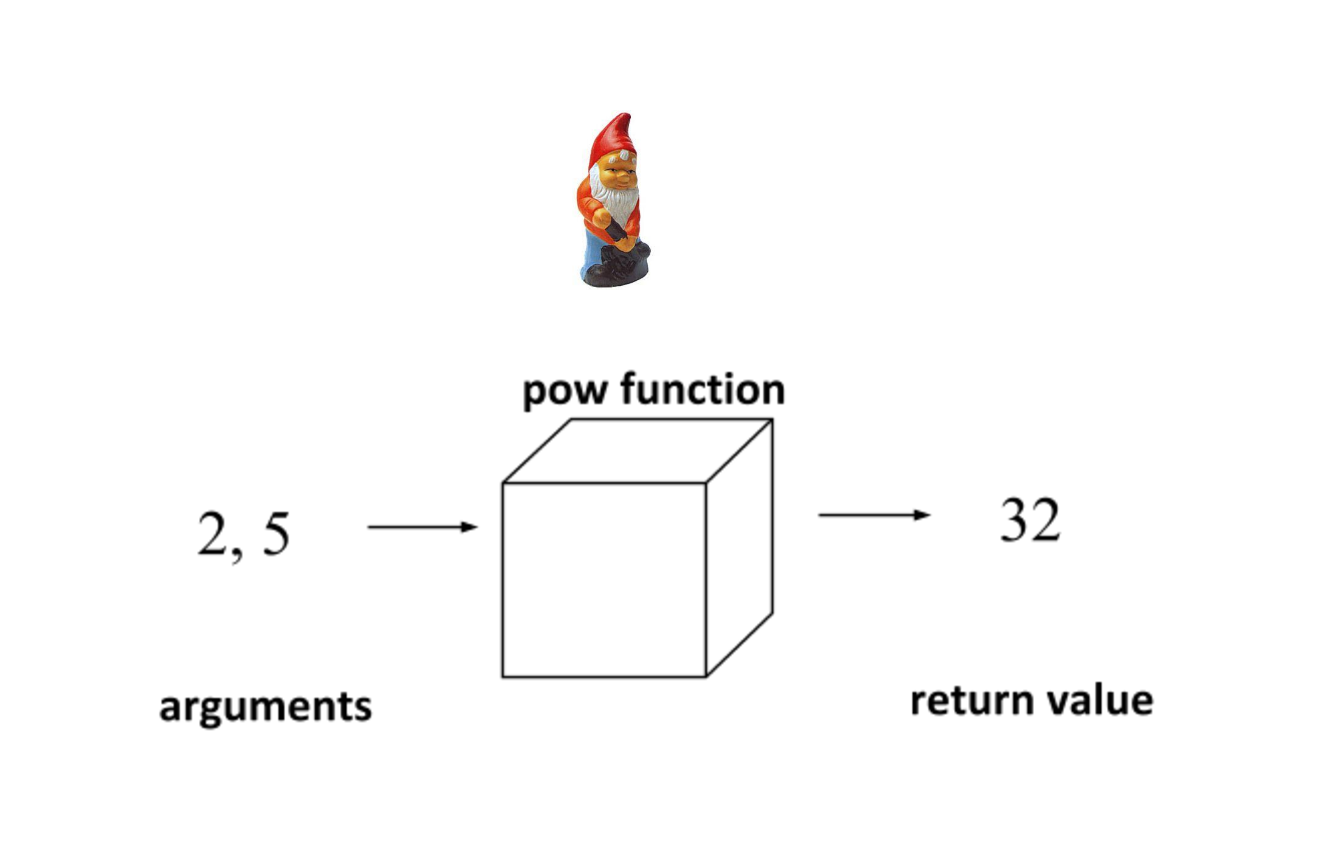## Side-effects and input¶

One thing that makes functions in Python different from the functions in your calculator or math book is that a function in Python can do more than just return a value. It can also affect the outside world, for example, by printing text in the shell, displaying a picture, or playing sounds. An action performed by a function in addition to returning a value is called a side-effect.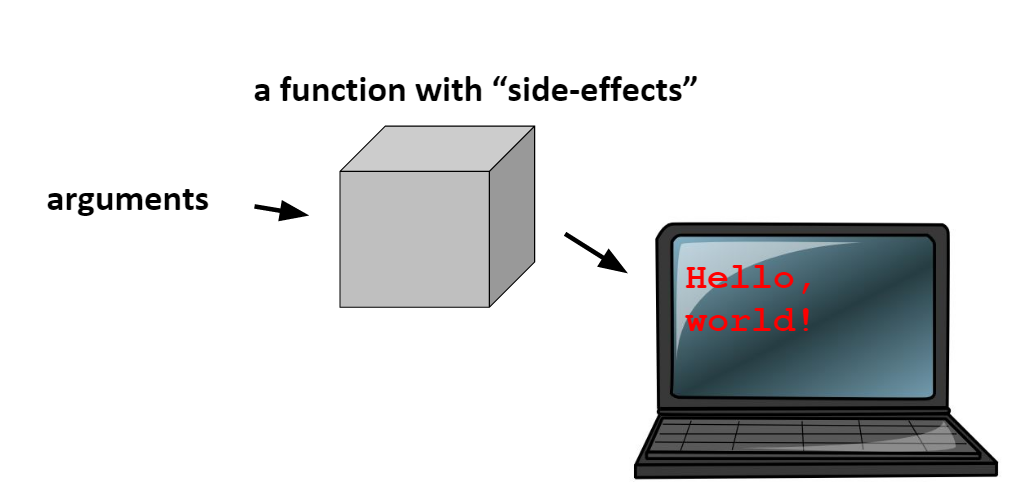We have already seen one example of a function with a side-effect: our friend the print function. Python has a function for reading input entered at the keyboard, helpfully called input. This is also a function with side-effects. It takes one argument, a string which we will call the prompt. When you call input, the interpreter:

displays the prompt on the screen,

waits for you to type something and press the ENTER key, and then

returns what you typed as a string

When you use input in an activecode window running in a browser, you don’t actually enter the text in the same window as the output. Instead, the browser will pop up a dialog box for you to type the input. Try this:

The prompt is actually optional, but in general you always want to include one. Otherwise the interpreter will just stop and wait without giving any clue to the user about what she’s supposed to do.

The input function always returns a string, even if you enter numbers:

Does this matter? Well, what if we want to do some arithmetic with a number entered by the user? Let’s try it:

This error is telling us that we can’t use the plus operator to combine an int with a string. In order to do this, we have to get the numeric value represented by the string result. There is a built-in function called int() for doing this. The return value of the int function is always of type int.

With this function, the string “42” was converted to an int, and can now be used in math (5 + x).

There is a similar function called float that converts a string such as “3.14” into a floating-point value.

So what happens if we give the int function something that isn’t a valid number at all?

Now, lets try input and int() together.

By using the int() function on result, it is converted to an int and can now be used in math.

## Writing an interactive script¶

You are probably accustomed to interacting with applications on your computer using a graphical user interface, or “GUI”, that has windows, buttons, or other features you can control with a mouse. Writing graphical interfaces is a bit beyond the scope of this course, but we can still create interactive applications with a text-based user interface. That is, we can read input from the keyboard and print textual output on the screen. An application with a text-based interface is traditionally called a console application.

Here is a simple example of an interactive script. We will read the user’s name and age, and then display a message showing what the user’s age would be in dog years, where one dog year is roughly 7 people-years.

We are using a special feature of the print statement, which is that we can print multiple values on one line if we separate them with a comma. Notice in the last line of our script we are actually printing three things:

The string “In dog years you are only”

The variable dog_years

The string “!”

Is there some way to get rid of the space between the years and the exclamation point? We can’t do it just using print statements like this. We will need one new idea.

## String concatenation and the str function¶

Programmers use the big word “concatenate” to describe the simple operation of combining several strings into one string. In Python, you use the plus operator for string concatenation.

You can see that if you want a space in the new string, you have to put one there yourself:

Let’s try an example. Say we’d like to print a dollar sign just to the left of a price. Using a comma in a print statement will leave a space:

What if we use concatenation?

What if we use concatenation?

It turns out that we can use a plus sign between two numbers to add them, or we can use a plus sign between two strings to concatenate them, but we can’t ever use a plus sign between a string and a number. We can only concatenate a string with another string.

Here there is a simple solution: we use a built-in function called str to convert x from a number to a string.

## Importing functions from a module¶

Python has a few built-in functions like len, pow, and input, but also has hundreds more that are in modules forming the “standard library”. For example, there is a module called math that has a function called sqrt for computing the square root. What’s the difference between a built-in function and a function from a module? In order to use the sqrt function, we would have to import it from the math module. You can try this right in the shell or in activecode:

When writing a script, you usually just put the import statements at the top of the file.

Most modules, like the math module, contain more than one function. To import more than one function from a module, you can use multiple import statements, or you can use one import statement and separate the function names with a comma. For example, suppose we want to use the cosine function in addition to the square root function. Then the import statement would look like this:

You can import ALL the functions in a module using a “wildcard”:

from math import *

However, that is not usually a good idea. There is an alternate form for the import statement that is a better choice when you want access to several functions from a module. Instead of importing functions by name from the module, you can just import the module. Then you can use any function in the module, as long as you precede it by the module name and a dot.